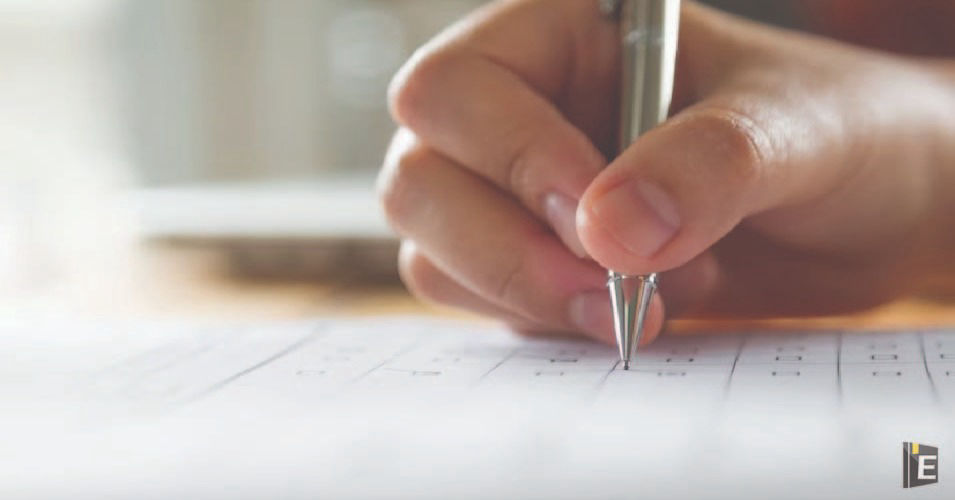• Save

# HCF and LCM Questions for Bank Exam

NEWFor bank exam aspirants, mastering concepts like Highest Common Factor (HCF) and Least Common Multiple (LCM) is crucial. These topics HCF and LCM Questions are frequently tested in quantitative aptitude sections of various bank exams. Understanding the principles behind HCF and LCM and employing efficient strategies to solve related questions can significantly boost one's chances of scoring well in these exams.

## HCF and LCM Questions

In this blog HCF and LCM Questions for Bank Exams, we will delve into the key concepts of HCF and LCM and provide valuable tips and tricks to tackle such questions with ease. If you are preparing for Bank Exams then this blog HCF and LCM Questions for Bank Exams will be very helpful for you.

Also, Read Latest Current Affairs Questions 2023: Current Affairs Today

"Put your knowledge to the test and excel with our General Knowledge Mock Test and Current Affairs Mock Test!"

## HCF and LCM Questions for Bank Exam

Q :

The greatest four digit number which is exactly divisible by each one of the numbers 12, 18, 21 and 28.

(A) 9828

(B) 9882

(C) 9928

(D) 9288

Q :

A number between 1000 and 2000 which when divided by 30, 36 and 80 gives a remainder 11 in each case is:

(A) 11523

(B) 1451

(C) 1641

(D) 1712

Q :

The number between 4000 and 5000 that is divisible by each of 12, 18, 21 and 32 is

(A) 4203

(B) 4023

(C) 4032

(D) 4302

Q :

The LCM of four consecutive numbers is 60 The sum of the first two numbers is equal to the fourth number. What is the sum of four numbers?

(A) 17

(B) 14

(C) 21

(D) 24

Q :

Two pipes of length 1.5 m and 1.2 m are to be cut into equal pieces without leaving any extra length of pipes. The greatest length of the pipe pieces of same size which can be cut from these two lengths will be

(A) 0.13 m

(B) 26 m

(C) 0.3 m

(D) 0.41m

Q :

The HCF and the LCM of two numbers are 5 and 120, respectively. If the sum of the two numbers is 55, then the sum of the reciprocals of these two numbers is equal to:

(A) $${{55}\over{601}}$$

(B) $${{11}\over{120}}$$

(C) $${{120}\over{11}}$$

(D) $${{601}\over{55}}$$

Q :

The LCM of two positive integers is twice the larger number and the HCF of the two numbers is 3. The smaller number is:

(A) 6

(B) 10

(C) 9

(D) 8

Q :

LCM of two numbers is 56 times their HCF, with the sum of their HCF and LCM being 1710. If one of the two numbers is 240, then what is the other number?

(A) 1680

(B) 171

(C) 210

(D) 57

Q :

The LCM of 1.2 and 2.7 is:

(A) 5.4

(B) 10.8

(C) 1.08

(D) 32.4

Q :

Find the HCF of 60, 148 and 382.

(A) 6

(B) 24

(C) 4

(D) 2